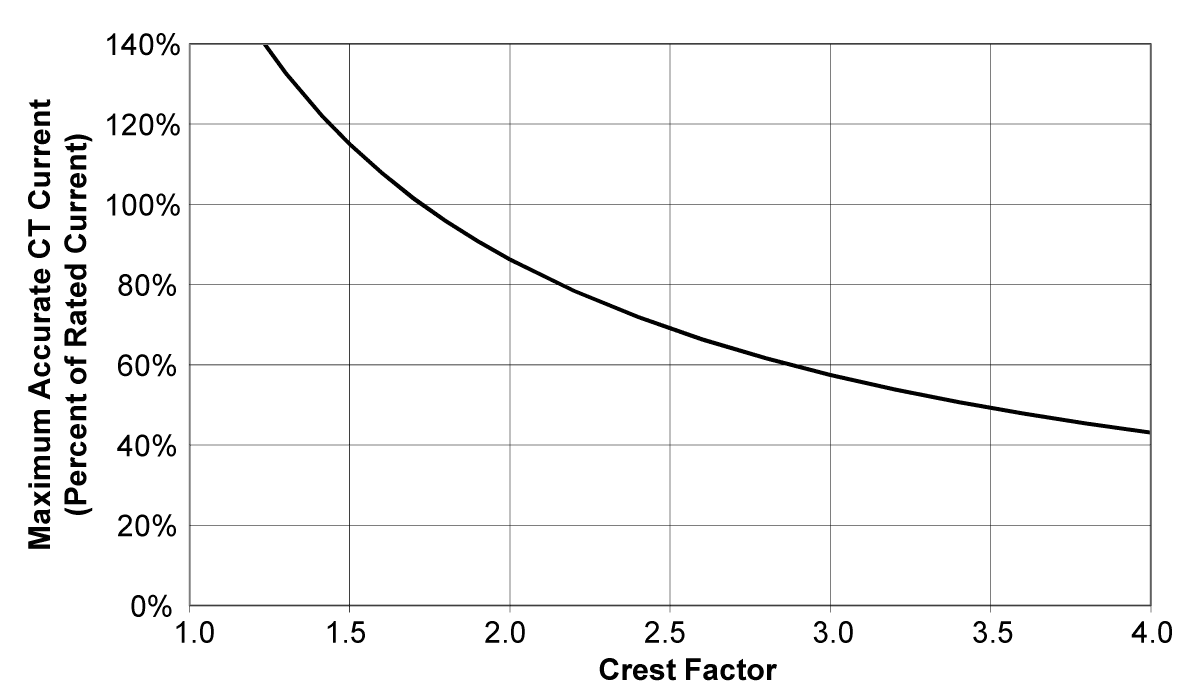The term “current crest factor”  is used to describe the ratio of the peak current to the RMS current (the RMS current is the value reported by multimeters and by the WattNode meter). Resistive loads like heaters and incandescent lights have nearly sinusoidal current waveforms with a crest factor near 1.4. Power factor corrected loads such as electronic lighting ballasts and computer power supplies typically have a crest factor of 1.4 to 1.5. Battery chargers, VSD motor controls, and other nonlinear loads can have current crest factors ranging from 2.0 to 3.0, and even higher.

High current crest factors are usually not an issue when metering whole building loads, but can be a concern when metering individual loads with high current crest factors. If the peak current is too high, the meter’s CT inputs can clip, causing inaccurate readings.

This means that when measuring loads with high current crest factors, you may want to be conservative in selecting the CT rated current. For example, if your load draws 10 amps RMS, but has a crest factor of 3.0, then the peak current is 30 amps. If you use a 15 amp CT, the meter will not be able to accurately measure the 30 amp peak current. Note: this is a limitation of the meter measurement circuitry, not the CT.

The following graph shows the maximum RMS current for accurate measurements as a function of the current waveform crest factor. The current is shown as a percentage of CT rated current. For example, if you have a 10 amp load with a crest factor of 2.0, the maximum CT current is approximately 85%. Eighty-five percent of 15 amps is 12.75, which is higher than 10 amps, so your measurements should be accurate. On the other hand, if you have a 40 amp load with a crest factor of 4.0, the maximum CT current is 42%. Forty-two percent of a 100 amp CT is 42 amps, so you would need a 100 amp CT to accurately measure this 40 amp load.You may not know the crest factor for your load. In this case, it’s generally safe to assume the crest factor will fall in the 1.4 to 2.5 range and select CTs with a rated current roughly 150% of the expected RMS current. So if you expect to be measuring currents up to 30 amps, select a 50 amp CT.